amortization.com Ltd.
Burlington, Ontario

905-639-0374
905-407-7988

info@amortization.comAnnual Percentage Rate (APR)

When arranging a mortgage or a loan there are other costs that increase the annual interest rate. In order to intelligently compare different scenarios from lenders you should not necessarily accept the lender with the lowest annual interest rate because of other costs. The Total Cost of Borrowing(TCOB) is a method used to compare various mortgages and loans. The TCOB is a percentage number that considers all the costs and their impact on the actual annual interest rate you will be paying.

These other costs can be summarized as follows.

1… UP FRONT COSTS such as Brokerage fees, finders fees, points or mortgage insurance.
2… CONSTANT COSTS such as monthly mortgage insurance premiums.
3… VARIABLE COSTS as declining insurance premiums or different fixed interest rates for fixed terms.
4… BACK END COSTS such as closing out fees, discharge fees charged by the lender to register the mortgage in the land registry office when the loan is fully amortized.

All costs must be converted to UPFRONT COSTS before you calculate the TCOB. The upfront costs are assumed to be paid in cash or by cheque, and thus treated as a seperate issue from the actual loan. In Canada the total cost of borrowing is quoted as the new effective interest rate (EIR) and then referred to as the TCOB. In the USA the total cost of borrowing is quoted as the new annual interest rate (AIR) and then referred to as the APR. The simplest total cost of borrowing calculation is showing total upfront costs spread over the entire amortization period as shown below. All TCOB calculations are made to fit this format by utilizing present value future value calculations. Let us assume the total costs are \$3000 for a \$100,000 loan at 4.7503% using “monthly compounding” amortized for 30 years.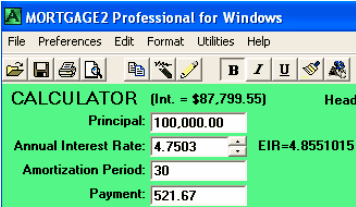Subtract the \$3000 from the \$100000 and recalculate the Annual Interest Rate for a \$97,000 loan by either left clicking on Annual Interest Rate prompt or left clicking on the Annual Interest Rate box and then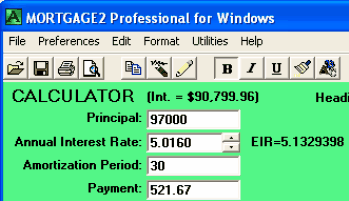pressing F6 . In the USA, the total cost of borrowing is shown by the new APR of 5.0160% assuming the loan utilized “monthly compounding”. In Canada the total cost of borrowing (TCOB) for this example would have been quoted as the new effective interest rate (EIR) of 5.1329%. The advantage of quoting the new EIR, the type of compounding does not need to be mentioned.

APR based upon up front costs
Let us assume the total costs amounted to \$3000 on closing day for a \$100,000 mortgage at 6% for 25 years using monthly payments with monthly compounding.

(Screenshot 1)

Subtract the \$3000 from the principal and recalculate on the Annual Interest Rate and the result is shown below;

(Screenshot 2)

The APR is 6.3236 % over the 25 years due to these upfront fees.

APR based upon future costs
QUESTION: What if the borrower had monthly insurance premiums of 20 dollars per month for the next 25 years. How would that be handled?

Enter a monthly payment of \$20 for 25 years at 6% and let the CALCULATOR show you what you could borrow. The Principal of \$3,104.14 is really the present value of a series of \$20 monthly payments over the next 25 years at 6% using monthly compounding.

(Screenshot 3)

Screenshot 4 is the negative amortization schedule showing that the Present Value/Future Value calculator agrees with the negative schedule (within pennies) as it should.

(Screenshot 4)

Subtract the \$3,104.14 from the Principal and recalculate on the Annual Interest Rate and the APR becomes 6.3351% as shown below.

(Screenshot 5)

APR based upon variable future costs

QUESTION: What if the borrower had monthly insurance premiums that were not constant but varied in the future?

For example, using an 8%, 30 year amortized mortgage with monthly payments and monthly compounding on an amount of \$100,000 as shown below.

(Screenshot 6)

The first 10 years the monthly insurance premiums would be \$81.67 and the next five years the premiums would be 16.67 per month.

The present value of these two insurance premiums future cash flows must be calculated using 8% as the interest rate. Using the "3 out of 4 feature" in MORTGAGE2 PRO CALCULATOR we pretend we are going to borrow money at 8% for 10 years with monthly payments of 81.67 and the Principal will automatically be calculated as \$6,731.36 which is the Present Value of that 10 year future cash flow.

(Screenshot 7)

Now do the same for monthly insurance premiums of \$16.67 but we must also do a PV-FV calculation to bring the \$822.14 further back ten years to the present.

(Screenshot 8)

The Present Value of the cash flows of the two insurance premiums is 6,731.36 + 370.40 = \$7,101.76 and using the discounting program,

(Screenshot 9)

the APR is 8.93% based upon 15 years (The % Yield you want). As can be seen below the APR based upon the full 30 years is 8.8% and that was easily calculated by pressing the up arrows and increasing the Discount Term to 360 months.

(Screenshot 10)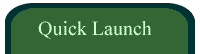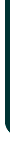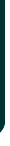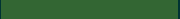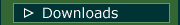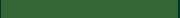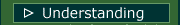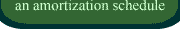VIDEOSamortizationdotcom Mortgage Calculator for iPhone

Introduction to Canadian and American Mortgages

Seminar on prepaying principal (Part A)

Seminar on prepaying principal (Part B)

Global TV Interview regarding 40 Year MortgagesLook for this logo on the Apple Store!

 < Go Back